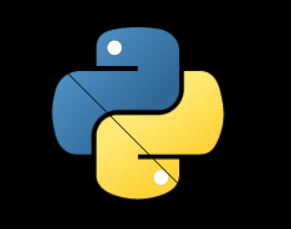GeeksforGeeks App
Open AppBrowser
Continue

# Python PIL | putpixel() method

PIL is the Python Imaging Library which provides the python interpreter with image editing capabilities. The PixelAccess class provides read and write access to PIL.Image data at a pixel level.
Accessing individual pixels is fairly slow. If you are looping over all of the pixels in an image, there is likely a faster way using other parts of the Pillow API.

putpixel() Modifies the pixel at x, y. The color is given as a single numerical value for single band images, and a tuple for multi-band images

Syntax: putpixel(self, xy, color)

Parameters:

xy :The pixel coordinate, given as (x, y)
value: – The pixel value.

Returns: An Image with pixel .

Image Used:`  ` `# Importing Image from PIL package ``from` `PIL ``import` `Image``     ` `# creating a image object``image ``=` `Image.``open``(r``'C:\Users\System-Pc\Desktop\python.png'``) `` ` `width, height ``=` `image.size`` ` `for` `x ``in` `range``(height):``    ``image.putpixel( (x, x), (``0``, ``0``, ``0``, ``255``) )`` ` `image.show()`

Output:Another example:Here we change the color parameter.
Image Used`# Importing Image from PIL package ``from` `PIL ``import` `Image``     ` `# creating a image object``image ``=` `Image.``open``(r``'C:\Users\System-Pc\Desktop\ybear.jpg'``) `` ` `width, height ``=` `image.size`` ` `for` `x ``in` `range``(height):``    ``image.putpixel( (x, x), (``10``, ``10``, ``10``, ``255``) )`` ` `image.show()`

Output:My Personal Notes arrow_drop_up